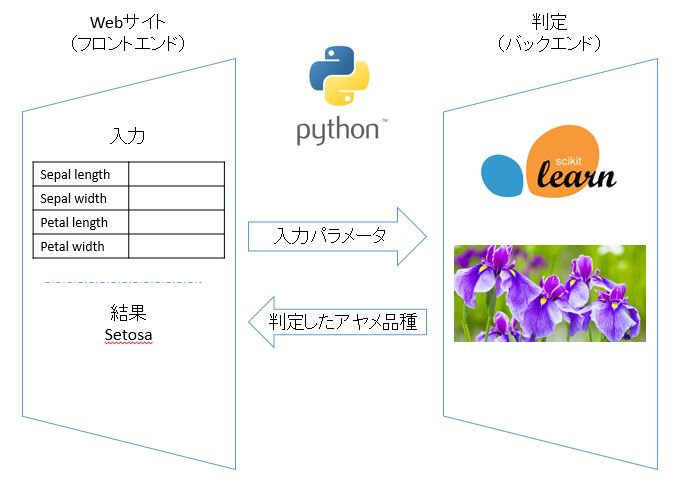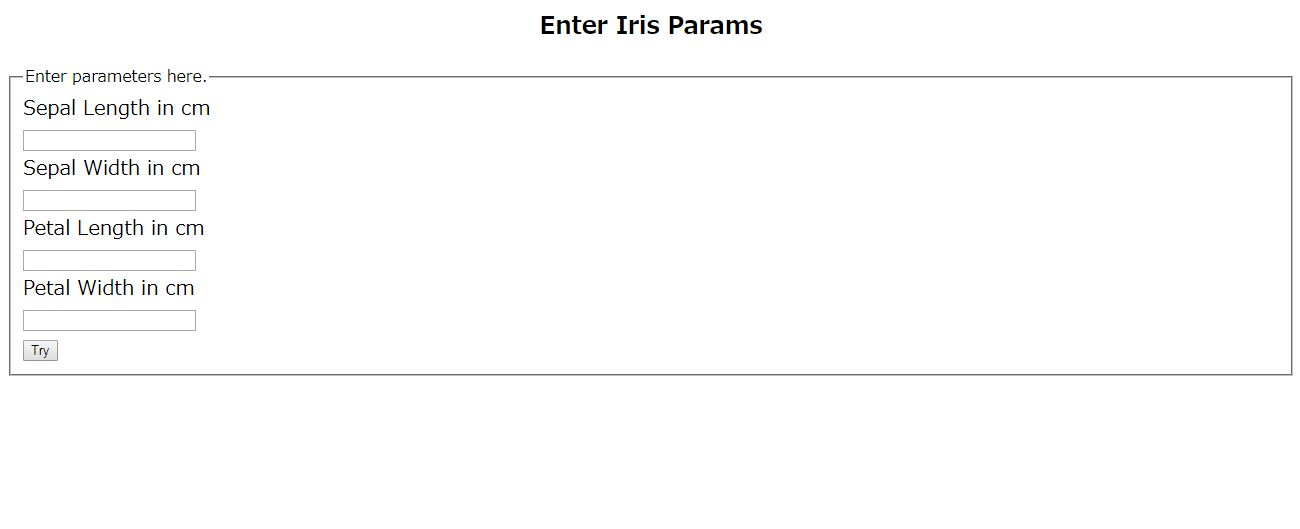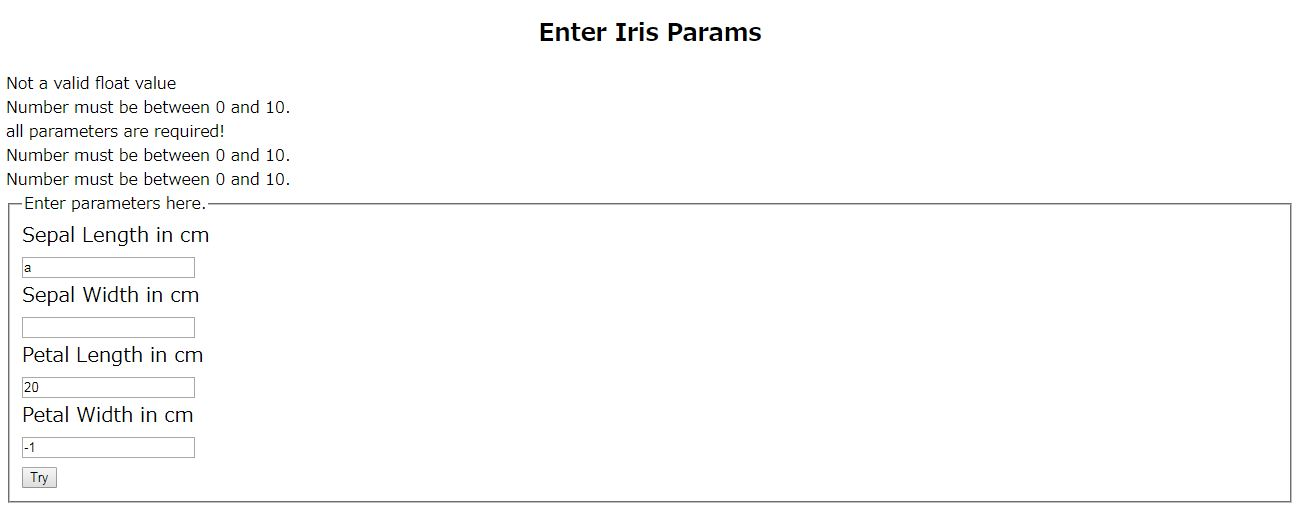# Simple machine learning and web apps

I made a simple demo app that displays the result on the screen with the judgment model created by machine learning for the input from the website. Enter the size of iris sepals and petals (vertical, horizontal) to determine and display the variety.

The code is here. https://github.com/shibuiwilliam/mlweb

## Overall picture

It is like this.It is composed of a back end that determines that it is a front end for inputting sepals and petals and returns the result. It's super simple. The language is Python 3.6, using flask and wtform for the web and scikit-learn for machine learning.

## How to use

The development environment is CentOS 7.3 and Python 3.6. The library includes flask, wtform, scikit-learn, and Jupyter Notebook. This area is mostly introduced in Anaconda3, but flask and wtform are pip installed. Please git clone the code.

``````
git clone https://github.com/shibuiwilliam/mlweb.git
``````

The website will be published on port 5000. It is necessary to set so that the OS and network can access 5000. (OS is selinux and firewalld)

Here's how to run the website.

``````
nohup python web.py &
``````

Execution logs are spit out to nohup.out. If you want to see the log sequentially, do the following:

``````
tail -f nohup.out
``````

## Machine learning

The iris judgment model uses scikit-learn's random forest and GridSearchCV.

Get the iris dataset provided by scikit-learn and create the model parameters with GridSearchCV along with the hyperparameters of the random forest.

``````
# iris_train.ipynb

from sklearn.datasets import load_iris
from sklearn.ensemble import RandomForestClassifier
from sklearn.model_selection import GridSearchCV, train_test_split
from sklearn.externals import joblib
import numpy as np

# use Iris dataset
x = data.data
y = data.target
x_train, x_test, y_train, y_test = train_test_split(x, y, test_size=0.3, random_state=54321)

# run gridsearchcv on random forest classifier
forest = RandomForestClassifier()
param_grid = {
'n_estimators'      : [5, 10, 20, 30, 50, 100, 300],
'random_state'      : ,
'n_jobs'            : ,
'min_samples_split' : [3, 5, 10, 15, 20, 25, 30, 40, 50, 100],
'max_depth'         : [3, 5, 10, 15, 20, 25, 30, 40, 50, 100]
}
forestGrid = GridSearchCV(forest, param_grid)
fgFit = forestGrid.fit(x_train, y_train)
``````

A model is created by fitting the created model parameters in a random forest. Save the model as a pickle. When used on a website, call the saved model.

``````
# set the best params to fit random forest classifier
forest.set_params(**fgFit.best_params_)
forest.fit(x, y)

# save the model as pickle
joblib.dump(forest, './rfcParam.pkl', compress=True)

# load the model

# predict
t = np.array([5.1,  3.5,  1.4,  0.2])
t = t.reshape(1,-1)
print(forest.predict(t))
``````

## Website

The Web is made with flask and wtform. First, define the functions used on the Web (web.py).

``````
# web.py

import numpy as np

#Save the input as a log in csv.
def insert_csv(data):
import csv
import uuid
tuid = str(uuid.uuid1())
with open("./logs/"+tuid+".csv", "a") as f:
writer = csv.writer(f, lineterminator='\n')
writer.writerow(["sepalLength","sepalWidth","petalLength","petalWidth"])
writer.writerow(data)
return tuid

# scikit-Judgment is made using the model created by learn.
def predictIris(params):
from sklearn.externals import joblib
# load the model
# predict
params = params.reshape(1,-1)
pred = forest.predict(params)
return pred

#Judgment is 0,1,Since it is output in 2, it is converted to the iris variety name.
def getIrisName(irisId):
if irisId == 0: return "Iris Setosa"
elif irisId == 1: return "Iris Versicolour"
elif irisId == 2: return "Iris Virginica"
else: return "Error"
``````

Below is the Python code using HTML and flask on the web.

First is the input form (templates / irisPred.html).

``````
<!doctype html>
<html>
<body>

<h2 style = "text-align: center;">Enter Iris Params</h2>

{% for message in form.SepalLength.errors %}
<div>{{ message }}</div>
{% endfor %}

{% for message in form.SepalWidth.errors %}
<div>{{ message }}</div>
{% endfor %}

{% for message in form.PetalLength.errors %}
<div>{{ message }}</div>
{% endfor %}

{% for message in form.PetalWidth.errors %}
<div>{{ message }}</div>
{% endfor %}

<form action = "" method = post>
<fieldset>
<legend>Enter parameters here.</legend>

<div style = font-size:20px; font-weight:bold; margin-left:150px;>
{{ form.SepalLength.label }}<br>
{{ form.SepalLength }}
<br>
{{ form.SepalWidth.label }}<br>
{{ form.SepalWidth }}
<br>
{{ form.PetalLength.label }}<br>
{{ form.PetalLength }}
<br>
{{ form.PetalWidth.label }}<br>
{{ form.PetalWidth }}
<br>
{{ form.submit }}
</div>

</fieldset>
</form>

</body>
</html>

``````

This is the screen (templates / success.html) that displays the judgment result.

``````
<!doctype html>
<title>Hello from Iris</title>
{% if irisName %}
<h1>It is {{ irisName }}</h1>
{% else %}
<h1>Hello from Iris</h1>
{% endif %}

``````

Python form definition (web.py).

``````
# web.py

from flask import Flask, render_template, request, flash
from wtforms import Form, FloatField, SubmitField, validators, ValidationError

# App config.
DEBUG = True
app.config.from_object(__name__)
app.config['SECRET_KEY'] = 'fda0e618-685c-11e7-bb40-fa163eb65161'

class IrisForm(Form):
SepalLength = FloatField("Sepal Length in cm",
[validators.InputRequired("all parameters are required!"),
validators.NumberRange(min=0, max=10)])
SepalWidth = FloatField("Sepal Width in cm",
[validators.InputRequired("all parameters are required!"),
validators.NumberRange(min=0, max=10)])
PetalLength = FloatField("Petal Length in cm",
[validators.InputRequired("all parameters are required!"),
validators.NumberRange(min=0, max=10)])
PetalWidth = FloatField("Petal Width in cm",
[validators.InputRequired("all parameters are required!"),
validators.NumberRange(min=0, max=10)])
submit = SubmitField("Try")

@app.route('/irisPred', methods = ['GET', 'POST'])
def irisPred():
form = IrisForm(request.form)
if request.method == 'POST':
if form.validate() == False:
flash("You need all parameters")
return render_template('irisPred.html', form = form)
else:
SepalLength = float(request.form["SepalLength"])
SepalWidth = float(request.form["SepalWidth"])
PetalLength = float(request.form["PetalLength"])
PetalWidth = float(request.form["PetalWidth"])
params = np.array([SepalLength, SepalWidth, PetalLength, PetalWidth])
print(params)
insert_csv(params)
pred = predictIris(params)
irisName = getIrisName(pred)

return render_template('success.html', irisName=irisName)
elif request.method == 'GET':
return render_template('irisPred.html', form = form)

if __name__ == "__main__":
app.debug = True
app.run(host='0.0.0.0')

``````

## Execution image

Enter an appropriate number (between 0 and 10) in the input form on the screen and try.The name of the judged iris variety is displayed on the screen.Check for input errors.